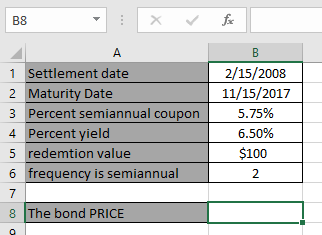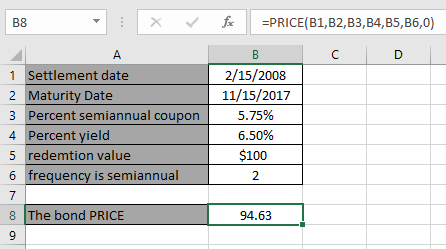# How to use the PRICE Function in Excel

The PRICE function is a built-in financial function which returns the price per \$100 face value of a security that pays periodic interest.
Syntax:

=PRICE(settlement, maturity, rate, yld, redemption, frequency, [basis])

Settlement : Settlement date of the security
Maturity : Maturity date of the security. The maturity date is the date when the security expires
Rate : The security's annual coupon rate
Yld : The security's annual yield
Redemption : Redemption value per \$100 face value
Frequency : Coupon payments per year (annual = 1, semiannual = 2; quarterly = 4.
basis : [optional] Day count basis

Let’s understand this function using it in an example.Here we have values to calculate the bond PRICE per \$100 face value of a security that pays periodic interest.
Use the formula:

=PRICE(B1, B2, B3, B4, B5, B6, 0)Note: Dates in the formula must be entered using cell reference. Excel date format creates problem while computing results.
Now Press Enter to get the PriceThe Price is \$ 94.63 .
As you can see the formula returns the price per \$100 face value of a security that pays periodic interest.

Hope you understood how to use PRICE function and referring cell in Excel. Explore more articles on Excel function here. Please feel free to state your query or feedback for the above article.

Related Articles:

Excel IPMT Function

How to use the DURATION Function in Excel

How to use the Excel IPMT Function

How to Use Compound Interest Function in Excel

Popular Articles:

50 Excel Shortcuts to Increase Your Productivity

How to use the VLOOKUP Function in Excel

How to use the COUNTIF in Excel 2016

Terms and Conditions of use

The applications/code on this site are distributed as is and without warranties or liability. In no event shall the owner of the copyrights, or the authors of the applications/code be liable for any loss of profit, any problems or any damage resulting from the use or evaluation of the applications/code.